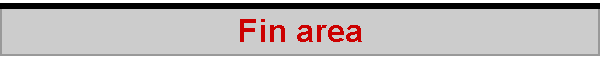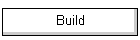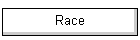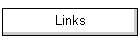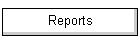By Larry Robinson, with permission.

One of the problems that you have to face in designing a new boat is how big to make the fin. A simple approach is to just copy other similar boats. However in some classes you will find a wide variation in area, with no good performance data to suggest what is best. (Of course you can always find lots of ideas about the subject!)

Another option is to use some sort of basic rule, such as making the fin five or some other percentage of the total sail area, etc. Such rules are not very satisfying because they don't address the variables involved in the function of the fin. Also, what might work for one class may not work for another.

Here is a different way of approaching the problem. I first used it to size the fins on a couple of small home-brew designs, then on a Marblehead project. Now, after ten years of procrastination, I'm finally writing it up! The M fin area calculation turned out to give essentially the same value that Bob Sterne had found over the years to be most appropriate. I guess that this might have been what persuaded Bob to try the formula on his AC design.

You may want to skip to the final fin area equation directly, but I'll go through the derivation in case you are interested.

Consider a boat sailing on the wind, going in a straight line, not speeding up or slowing down. The boat is at equilibrium. The net force on the boat, in spite of all the strain on different parts of the hull and rig, is zero! For every force affecting the boat's motion, there is an equal and opposite force. (That's why Ross Garrett named his book The Symmetry of Sailing.)Figure 1

In this light the function of the fin, besides supporting the ballast, is to oppose the side force generated by the sails. (Both Ross Garrett and Tony Marchaj have nice graphics in their books that illustrate this idea.) In other words, the wind, acting on the sails, produces a force that can be resolved as a vector parallel to the course (often called the driving force or thrust vector) and one perpendicular to the course. Let's call this latter vector the sail side force. (For simplicity, these vector names are slightly different than those used by Garrett or Marchaj.)

From the symmetry argument above, there must be equal and opposite vectors to those generated by the sails. These are supplied by the hull. The equal and opposite vector to 'thrust' is the drag of the hull. Since these two vectors are equal and opposite, there is no net force. Since force = mass x acceleration, if there is no net force, there is no acceleration. The boat speed is constant. That was the premise that with which we started.

Because we stipulated that the boat was not accelerating in any direction (even sideways) there must also be a vector equal and opposite to the sail side force. That vector is supplied by the hull (including the fin) and can be called the hull side force. See Figure 1.

This can be written as:

Eq. 1         Fhull side force = Fsail side force

Notice that the hull side force vector (Fhull side force) like the sail side force vector is perpendicular to the course. It's perpendicular to the flow of water, not the direction the boat is pointed. Such a force, perpendicular to the flow, meets the definition of lift. (Also, the force parallel to the flow is defined as drag.) See Figure 2. So the hull side force is just the lift created by the hull/fin combination.Figure 2

Bodies such as the hull or fin create lift by operating at an angle of attack. In the case of a symmetrical foil such as a hull or fin, the angle of attack is the angle between the centerline of the foil and the flow. On a sailboat, this is approximately the same as the leeway angle. It's not exactly the same. Think of what happens during a knockdown, when the heel angle is about 90 degrees and the boat is skidding sideways! The leeway is closing in on 90 degrees but the angle of attack is about zero. Without proof, I'll suggest that the leeway angle times the cosine of the heel angle = angle of attack.

It would be nice if the fin alone could supply all of the side force (lift) because it's a more efficient shape. However, unless the fin can be pivoted a bit (like a rudder) the hull will also go through the water at some small angle of attack. So it will produce lift too. Bob Sterne gave me an eyeball estimate that the fin of an M produces about 90% of the required lift, while the hull supplies 10%. These values will be used later. As an aside, notice what happens when the fin stalls. Maybe these percentages are reversed! Not good.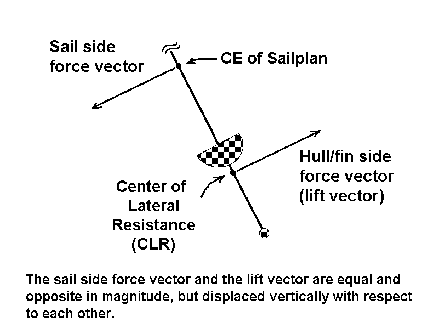Figure 3

Again let's go back to the 'symmetry of sailing' and ask about the forces having to do with heeling. Since the boat was presumed to be at equilibrium, the heel angle is not changing. This means that the torque trying to heel the boat is equal and opposite to the torque trying to keep it upright. (Torque is a force times the distance to an axis of rotation.) This leads to the following:

Eq. 2         torque produced by the sails = torque produced by the hull

It might appear at this point that the force generated by the lift of the fin (which adds to heeling) is being neglected. I don't think that is the case, based on the method of computing torque to follow.

Looking at equation 2, you can see that the term 'torque produced by the hull' is, by definition, the righting moment. Without going into the derivation here, righting moment (RM) can be expressed as the following:

Eq 3         RM = displacement x metacentric height x sin (heel angle).

Substituting, we get:

Eq. 4        torque produced by the sails = torque produced by the hull = RM
= displacement x metacentric height x sin (heel angle).

Now we need to find the torque produced by the sails. See Figure 3. By the symmetry argument, there is a side force generated by the hull/fin which is equal and opposite to the side force on the sail. If this were not so, the boat would be accelerating sideways. These two force vectors are parallel, however they are displaced with respect to one another. If two forces are equal and opposite, and displaced, the torque is measured by the product of one of the forces times the distance between them. (This is called a couple.) So we don't need both the force on the sails and the force generated by the hull/fin. Only one is needed.

Since we are considering fin area, let's use the side force generated by the hull/fin. Remember that this force, perpendicular to the flow, is just lift.

We now have, with a little substitution:

Eq 5         torque from the sail
= hull/fin side force (lift) x the distance between the hull fin force and the sail force

The distance from the hull/fin force and the sail force can be expressed as the distance from the center of effort (CE) of the sail plan to the center of lateral resistance (CLR) of the hull/fin. (This comes from the definition of these terms.)

Rewriting, this gives:

Eq. 6         Torque from sail (including the contribution from the lift of the fin)
= lift force of the hull/fin x (CE to CLR)

Remembering that the torque from the sails equals the torque from the hull and that the torque from the hull is its righting moment, we get:

Eq. 7         Torque by hull = Disp. x metacentric height x sin heel angle
= lift force of the hull/fin x (CE to CLR)

I should mention a slight simplification here. The torque produced by the hull (righting moment) acts in relation to the centerline of the boat, not the angle of flow from which the vectors were referenced. Since this is only a 3 to 5 degree error, it is neglected here.

Solving Equation 7 for the lift of the fin/hull gives:

Eq. 8         Hull/fin lift = (Disp. x metacentric height x sin heel angle) / (CE to CLR)

At the beginning we estimated that the lift of the fin = .9 x hull/fin lift. So the lift generated by the fin is:

Eq. 9         Lift from fin = (0.9 x Disp. x metacentric height x sin heel angle) / (CE to CLR)

Assume now that you are designing a boat with a relatively high aspect ratio fin. Maybe a M or a One Meter. In this case, tip losses can be neglected, at least for what is to follow. From 'lifting line theory' (which is used to predict lift) the lift coefficient seen in airfoil handbooks is defined as:

Eq. 10         Coefficient of lift
= (9.81 x lift force, measured in kg) / (˝ fluid density x velocity2 x surface area)

The units here must be kilograms, meters, seconds. (The MKS system.) The value 9.81 is there to convert force, which is measured in newtons, to kilograms. That way the units work out.

A brief explanation: force = mass times acceleration. On the surface of the earth, gravitational acceleration is 32 feet per second per second, on in the metric system, 9.81meters per second per second. So a 1 kg mass produces a gravitational force (in newtons) of 1 x 9.81 = 9.81 newtons.

Notice that Equation 10 includes the surface area for the foil. That's what we are trying to find. It also includes the lift force generated by the fin, measured in kilograms. That same lift force is also found in Equation 9.

So by substitution, you can solve for fin area and rearrange the terms to give:

Eq. 11         Fin area (in square meters)
= (9.81 x 0.9 x displacement in kg x sin heel angle x metacentric height) /
(˝ fluid density x velocity squared x (CE to CLR) x lift coefficient of fin)

The units must all be in the MKS system. Fluid density can be taken as 1000 kg per cubic meter.

Expressed in equation form, the final result is:

Fin area =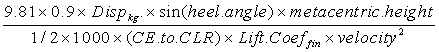Units: meters, kilograms, seconds. (Note: this equation does not account for tip losses, which are substantial with low aspect ratio planforms. Use it for high aspect ratio fins only.)

## How to use the fin area equation

The idea is to consider the fin as a foil, like an airplane wing, and decide in what range of lift coefficients you want it to operate. Think of the lift coefficient as a way to express the lift of a foil as the angle of attack changes, in a dimensionless way. With Equation 10, you can convert to actual forces. Depending on flow characteristics, which are specified here in terms of Reynolds numbers, there always is a maximum angle of attack above which the foil will stall. So there is a maximum lift coefficient too. So you already know from the equation that there will be a minimum fin size, below which the fin would be stalled all the time.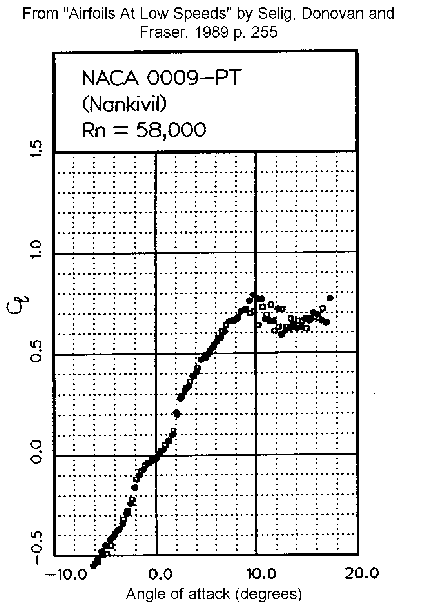Figure 4

Here is one way to go about choosing a reasonable lift coefficient to use in the formula. First select or define a 'design heel angle'. Let's say that at a design heel angle of 30 degrees, you want the lift coefficient to still be relatively low when the boat is up to speed. This way you have 'reserve lift capability' when you are going much slower, either after a tack, when sailing in waves, etc. You also need to have reserve lift for the times when the heel angle is too high.

You can now refer to plots of the lift coefficient vs. angle of attack. The classic Theory of Wing Sections by Abbott and Von Doenhoff has many such plots, but unfortunately they are not at suitable Reynolds numbers. Also, as I understand, there were turbulence factors not fully accounted for when the data was accumulated, such that the actual Reynolds numbers were significantly higher. Using lift coefficients for high Reynolds numbers will cause you to greatly overestimate what your foil can do at lower values.

(A simple formula for Reynolds number in water is Rn = speed in mph x chord in inches x 10,000. Think of this as a way to compare the characteristics of fluid flow. If the Reynolds number is the same, the character of the flow is the same whether the fluid is air, water or something else.)

My source for low speed foil coefficients is Airfoils at Low Speeds* by Selig, Donovan and Fraser. This book has data down to an Rn of 40,000 as compared to typical values from other sources, which are in the millions. This is important because if your fin has a 2 inch chord at the bottom at a speed of 2 mph, the Rn is still only 40,000. (40,000 is sometimes called a 'critical Rn' because the flow can be unpredictable below this value.)

An example of a lift plot for a NACA 0009 foil, taken from Airfoils at Low Speeds is shown in Figure 4. Plots at Reynolds numbers from 40,000 to 100,000 indicate that the maximum lift coefficient in this flow regime is about 0.7 to 0.8. The foil stalls at that point. Not considering tip effects, this corresponds to an angle of attack of about 10 degrees.

Compare this low lift coefficient with the value of 2.0 for the same foil at 6 million Rn (taken from Abbot and Von Doenhoff). If you used this value, you would make your fin way too small. Like maybe 2˝ times too small!

Now you can choose a lift coefficient at which you want your fin to operate. You might be tempted to select the value that corresponds to the maximum lift/drag. For the Rn values we are considering, this occurs at about a lift coefficient of 0.5. This choice would be way too high, as it would not give enough allowance for adverse conditions. Maybe an eyeball choice of 0.2 would be better. You might think that this value is way too conservative. However, the Reynolds number problem must be considered. For example, when you are coming out of a down-speed tack at one knot, a fin with a 2˝ inch chord is operating at an Rn of only 25,000. The flow may be very unpredictable. If the fin has a substantial chord, perhaps you could choose a higher lift coefficient. Say up to 0.3.

Also, you don't want the fin to stall when the heel angle goes up. If the heel angle increases from the nominal 30 degrees to say 60 degrees in a gust, your chosen lift coefficient will have to increase by a factor of 1.7 times. (This comes from the ratio of the sine of the heel angles.) Check the lift plot (Figure 4) to see if that is possible. In addition, this example assumes that your boat will not slow down at 60 degrees heel, which of course it will. Experience with the formula helps here.

You can also approach the choice this way. The relationship between angle of attack and the lift coefficient is about linear. For the Rn values appropriate for our models, an approximation taken from Figure 4 is:

lift coefficient x 12 = ~ angle of attack

Many modern (full size) sailboats make about 3 degrees leeway when sailing at a 'design heel'. From the equation cosine of the heel angle x leeway angle = angle of attack, the angle of attack at 30 degrees heel could be estimated at 3 x 0.86 = ~2.5 degrees.

This would correspond to a lift coefficient of about 3.5 / 12 or ~ 0.2. I'll use this value in the example to follow.

Now you only need a few more values in order to use the formula. You can time a few similar boats sailing on the wind (at your chosen heel angle) to get the speed. Sure, it's a squared function, so errors are magnified. But remember that the lift coefficient of your fin is always changing as you maneuver and as the weather changes. You are just trying to size the fin to keep the lift coefficient in a reasonable range most of that time.

You also need a value for the 'CE to CLR'. The vertical height of the center of effort of each sail can be taken as roughly 40% up the luff of each sail. Use a weighted average in relation to sail area to find the position of the CE of the combined sailplan. If you have access to a similar rig, here is a neat empirical way to measure the vertical location of the CE. Make a temporary support for the jib and main booms from a stick. Make sure the jib sheeting angle is appropriate. With your finger tips, grasp the rig by the mast and hold it up in the wind as if beating. Move your fingers up and down until the rig stays vertical. Mark the position, which corresponds to the vertical location of the CE. Minor errors are not critical here, as the distance to the CLR is large.

You can roughly estimate the CLR as the lateral center of area of the fin and hull. Since the fin produces most of the lift, this will be a bit high, but it's a small error in relation to the distance to the CE. The lateral center of area can be guesstimated by cutting out a cardboard profile of the hull and fin and balancing it on a ruler. (Omit the bulb, because it should not produce much lift.) Very rough, but adequate for the purpose. Now you can figure the distance between the CE and CLR.

The only value remaining to be determined is the metacentric height. This is the distance between the center of gravity and the metacenter at the chosen heel angle. See Figure 5. You can try to predict the center of gravity with a table of weights, or by getting approximate values from similar boats. If you do have access to a comparable boat, find and mark a point on its fin where it will balance horizontally, fully rigged. This will allow you to measure depth of the CG below the waterline.

Finding the metacenter can be done quite laboriously by traditional naval architecture methods, or perhaps if you have access, with the help of one of the specialized computer programs used for the purpose.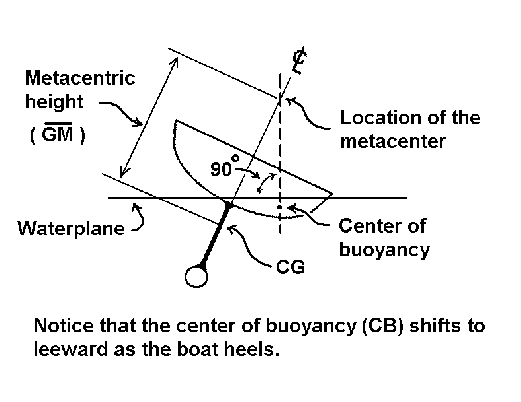Figure 5

The metacenter location can also be estimated empirically. Here's how: You need the canoe body of the boat only. No fin, bulb, rudder, interior equipment, etc. Prepare a rectangular 'brick' of some kind, uniform in density, and equal to the expected displacement of the boat, minus the weight of the canoe body. It need not be extremely precise. Place the brick in the hull with its long axis parallel to the centerline of the boat. Put the boat in the tub and slide the brick laterally until the boat heels to about 30 degrees. Measure the exact heel angle with a small bubble level and some clay. (Tape a temporary thwart across the boat. Stick a blob of modeling clay on it, then push a bubble level into the clay, centering the bubble. You can now duplicate the angle and measure it.)

Mark the exact position of the brick in the hull and also mark the heeled waterline on both sides of the hull. Make a duplicate of Figure 5, using the full scale section of your boat at maximum beam. From your measurements, draw the waterplane at the correct angle and location. Also draw in your 'brick' at the right location. (You may need to make a few more measurements to do this.)

We'll assume that the weight of the canoe body is but a small percentage of the total weight of your boat, ready to sail. Find the center of gravity of the brick, which represents most of the weight of the boat. (This makes the reason for using a brick shape clear. It's easy to find the CG.) As a close approximation, the center of buoyancy is directly under the CG of the brick, perpendicular to the heeled waterplane. Project a line upwards through the CG of the brick, again perpendicular to the heeled waterplane. (Refer to Figure 5.) The intersection of this projected line with the centerline of the boat is the empirically derived metacenter. Again, this is an inexact method. It neglects the weight of the canoe body, the buoyancy of the fin and rudder, etc.

Measure the distance from the metacenter to your predicted center of gravity and you have the metacentric height. You now have all the values needed to compute the fin area.

Here is an example of how to use the equation. These values are from an M design I did about 1987.

Displacement = 3.95 kg
Speed = 1.45 m/sec (2.0 knots, measured at ~30 degree heel)
"Design" heel angle = 30 degrees
Metacentric height = .412 meters
CE to CLR = 1.04 meters (A rig)
Target lift coefficient = .20 (based on being up to speed, 30 degrees heel and very narrow fin)

The formula again is:

Fin area =So,

Fin Area = ( 9.81 x 0.9 x 3.95 x .5 x .412) / (0.5 x 1000 x 1.04 x 0.2 x 1.45 x 1.45)
= 0.0328 square meters
= 50.9 square inches  (1 square meter = 1550 square inches)

Notice that there are two values whose choice is somewhat arbitrary; the lift coefficient and the heel angle. If we let the heel angle be 25 degrees instead of 30 degrees in the example above, the calculated fin area above would be 43 square inches. This would generally be regarded as a bit too small for an M. Likewise, the chosen lift coefficient of .20 could have been a different value, perhaps significantly changing the area. Nonetheless, the values selected (lift coefficient = 0.2 and heel angle = 30 degrees) give a reasonable result for fins with short chords. I suggest you start with these numbers unless experience dictates otherwise.

At this point it's clear that the formula is in some sense is just another arbitrary rule. However unlike a simple sail area to fin area rule, this method does address what the fin really has to do. Create lift. The amount of lift needed is a function of the righting moment (which includes the displacement) the height of the rig, the depth of the fin and of course the sail area (which is expressed in the formula as it relates to heel angle). All these things are accounted for in this method.

Larry Robinson 1-98

Thanks to Professor Michael S. Selig for permission to use the graphic shown in Figure 4.

*Airfoils At Low Speeds by Michael Selig, John Donovan and David Fraser, 1989. Published as SoarTech 8 by H. A. Stokely, SoarTech Publications, 1504 N. Horseshoe Cir., Virginia Beach VA 23451 U.S.A. (Email herkstok@aol.com)

2005-12-18